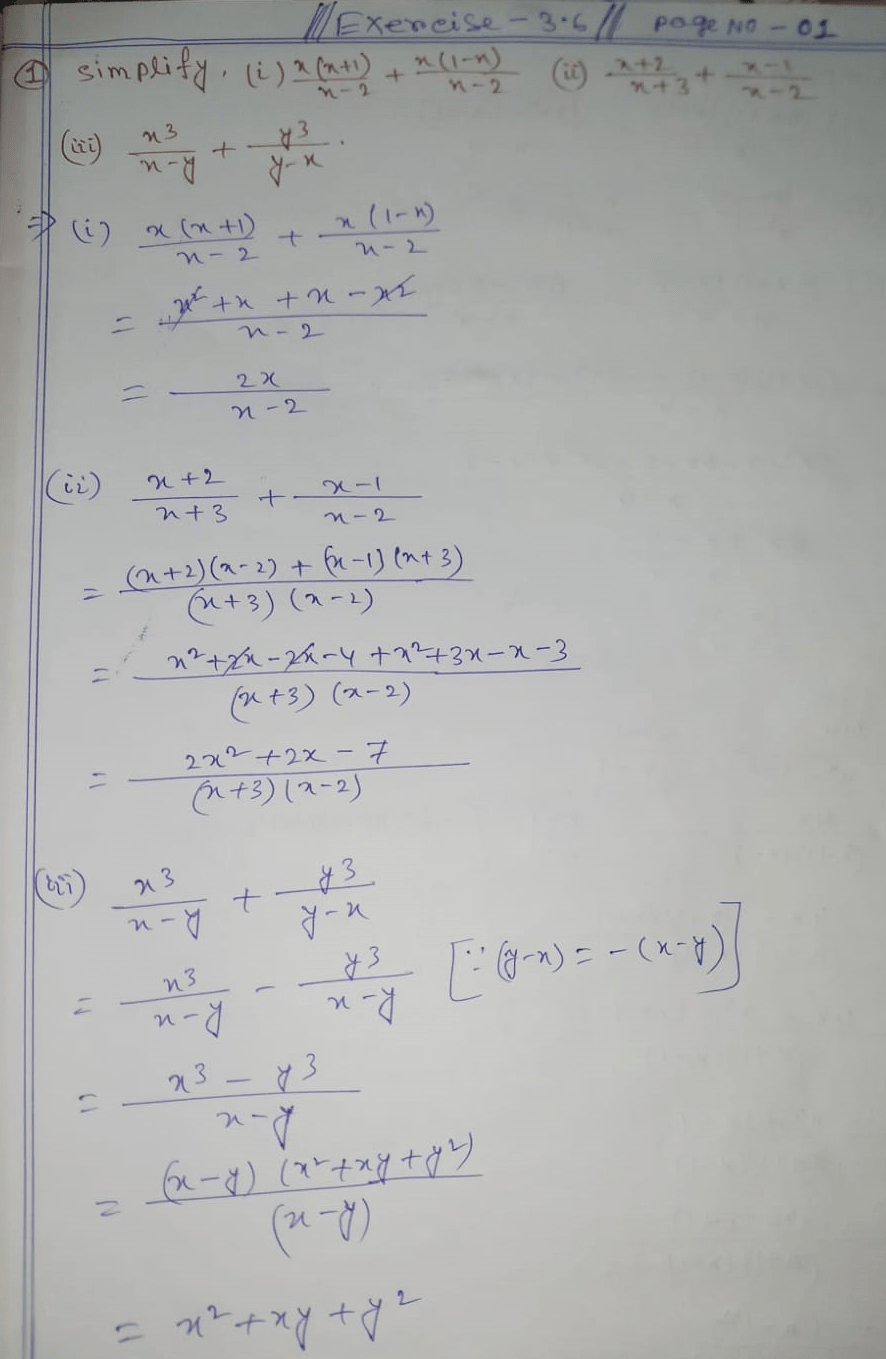# Samacheer Kalvi 10th Maths Solutions Chapter 3 Exercise 3.6 Pdf

## Samacheer Kalvi 10th Maths Solutions Chapter 3 Exercise 3.6 Pdf

TN Samacheer Kalvi 10th Maths Solutions Chapter 3 Exercise 3.6 : Hello students, Are You looking for Samacheer Kalvi 10th Maths Solutions Chapter 3 Exercise 3.6 – Algebra on internet. If yes, here we have given TNSCERT Class 10 Maths Solution Chapter 3 Exercise 3.6 Pdf Guide for Samacheer Kalvi 10th Mathematics Solution for Exercise 3.6

### TN Samacheer Kalvi 10th Maths Solutions Chapter 3 Exercise 3.6 – Algebra

 Board TNSCERT Class 10th Maths Class 10 Class Subject Maths Chapter 3 (Exercise 3.6) Chapter Name AlgebraHere we posted the solution of TN Tamilnadu Board Samacheer Kalvi 10th Maths Solutions Chapter 3 Algebra Exercise 3.6. TNSCERT Samacheer Kalvi 10th Maths Chapter 3 Exercise 3.6 Algebra Solved by Expert Teacher.

Updated: December 17, 2021 — 3:32 pm